GeeksforGeeks App
Open AppBrowser
Continue

## Related Articles

• RD Sharma Class 10 Solutions

# Class 10 RD Sharma Solutions – Chapter 7 Statistics – Exercise 7.6

### Question 1. Draw an ogive by less than the method for the following data:

Solution:

We plot the points (1, 4), (2, 13), (3, 35), (4, 63), (5, 87), (6, 99), (7, 107), (8, 113), (9, 118), (10, 120) respectively by taking the upper-class limit over the x-axis and cumulative frequency over the y-axis of the graph.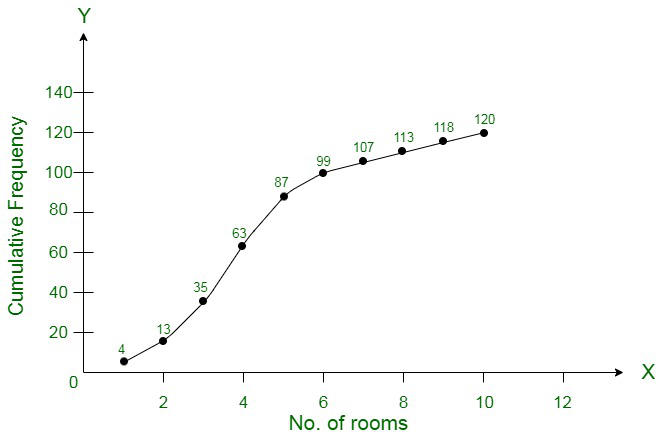### Prepare a cumulative frequency distribution table by less than method and draw an ogive.

Solution: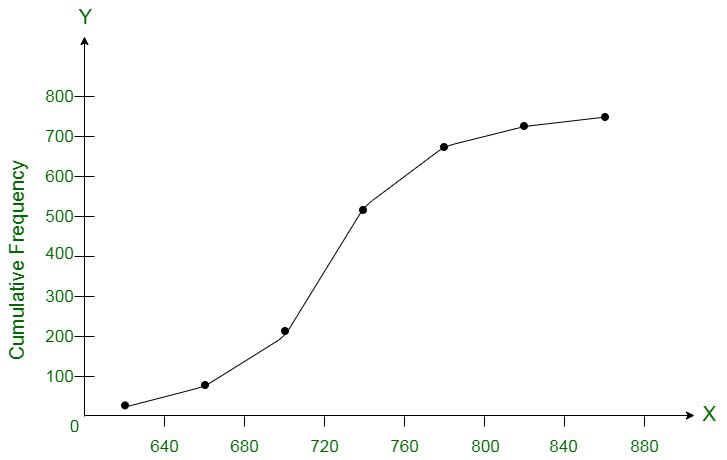### Question 3. Draw an Ogive to represent the following frequency distribution:

Solution:

Converting the given frequency distribution into continuous frequency distribution:

We plot the specified points (4.5, 2), (9.5, 8), (14.5, 18), (19.5, 23), (24.5, 26) on a graph by taking the upper class limit over the x-axis and cumulative frequency over the y-axis respectively.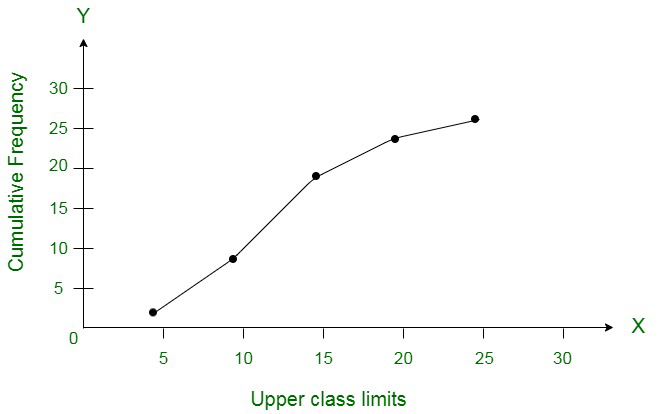### Draw the frequency polygon for it.

Solution:

Now, computing the following data, we have,

Now, the frequency polygon can be computed as follows :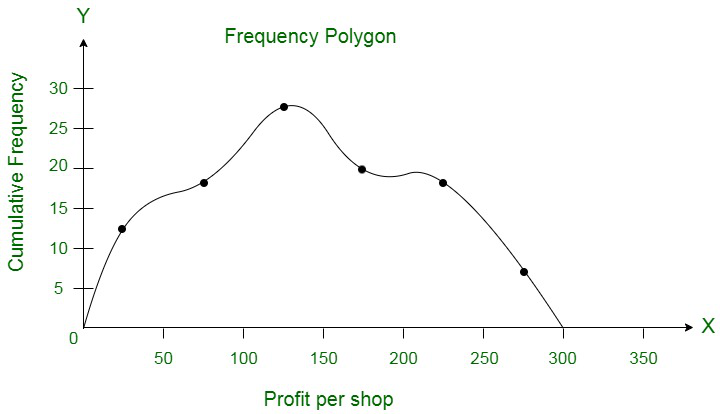### Convert the above distribution to a ‘less than’ type cumulative frequency distribution and draw its ogive.

Solution:

Using the less than method, the following distribution can be converted to a continuous distribution, as,

Mark the point (120, 12), (140, 26), (160, 34), (180, 40), (200, 50), taking upper class limit on the x-axis and cumulative frequencies on y-axis respectively.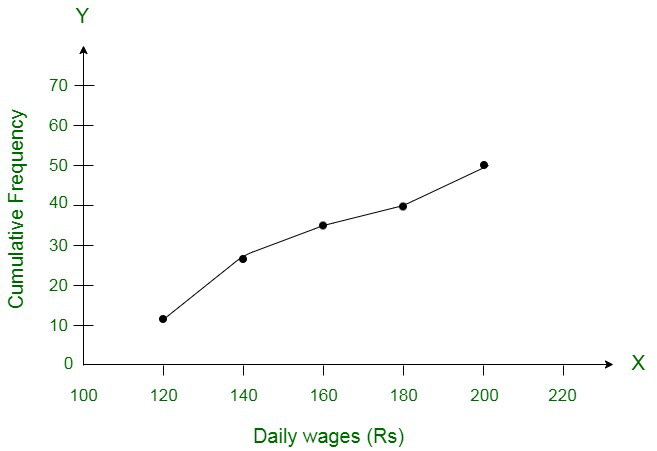### Draw ‘less than’ ogive and ‘more than’ ogive.

Solution:

(i) Computing the Less than ogive

We plot the specified points (55, 2), (60, 10), (65, 22), (70, 46), (75, 84) and (80, 100) and connect them to form an ogive.

(ii) More than

We plot the specified points (50, 100), (55, 84), (60, 46), (65, 22), (70, 10), (75, 2) and (80, 0) and connect to form a more than ogive as shown below: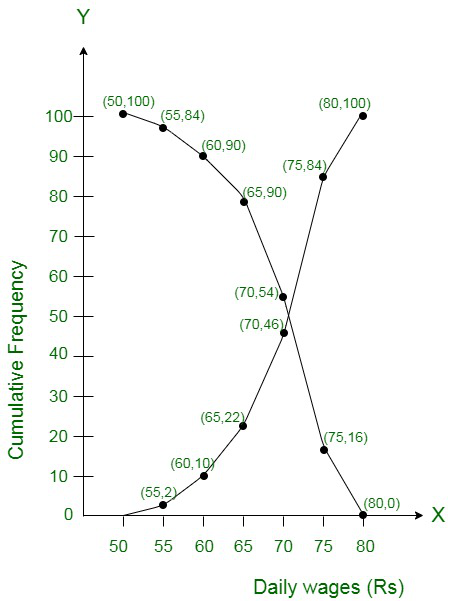### Draw a less than type ogive for the given data. Hence, obtain the median weight from the graph and verify the result by using the formula. (C.B.S.E. 2009)

Solution: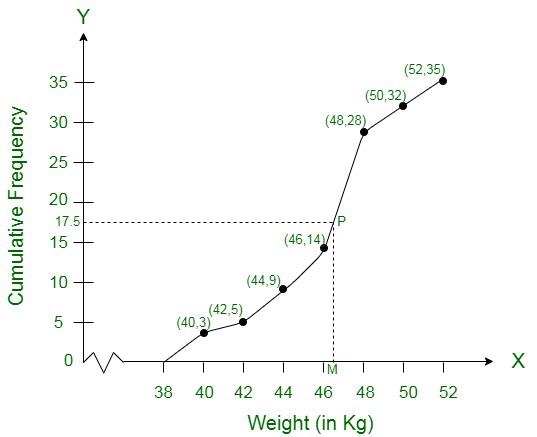Plot the points (38, 0), (40, 3), (42, 5), (44, 9), (46, 14), (48, 28), (50, 32), (52, 35) on the graph and join them in free hand to get an ogive as shown.

Here N = 35 which is odd

∴= 252 = 17.5

From 17.5 on y-axis draw a line parallel to x-axis meeting the curve at P. From P, draw PM ⊥ x-axis

∴ Median which is 46.5 (approx)

Now N = 17.5 lies in the class 46 – 48 (as 14 < 17.5 < 28)

∴ 46-48 is the median class

Here l= 46, h = 2,f= 14, F= 14

∴ Median =### Calculate the median rainfall using ogives of more than type and less than type. [NCERT Example]

Solution:

We observe that, the annual rainfall record of a city less than 0 is 0. Similarly, less than 10 include the annual rainfall record of a city from 0 as well as the annual rainfall record of a city from 0-10. So,

the total annual rainfall record of a city for less than 10 cm is 0 + 22 = 22 days. Continuing in this manner, we will get remaining less than 20, 30, 40, 50 and 60.Also, we observe that annual rainfall

record of a city for 66 days is more than or equal to 0 cm. Since, 22 days lies in the interval 0-10. So, annual rainfall record for 66 – 22 = 44 days is more than or equal to 10 cm. Continuing in this

manner we will get remaining more than or equal to 20, 30 , 40, 50, and 60.

Now, we construct a table for less than and more than type.

To draw less than type ogive we plot the points (0,0), (10,22), (20,32), (30, 40), (40, 55), (50, 60), (60, 66) on the paper and join them by free hand.

To draw the more than type ogive we plot the points (0, 66), (10, 44), (20, 34), (30, 26), (40, 11), (50, 6) and (60, 0) on the graph paper and join them by free hand.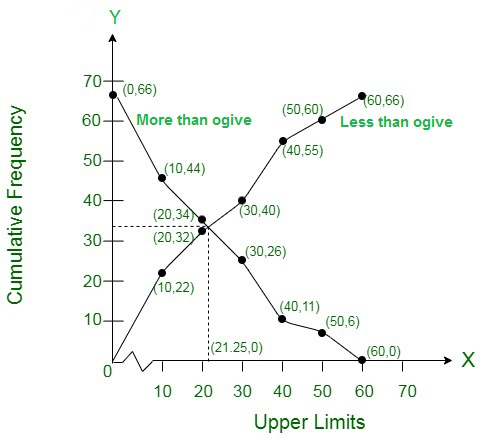∵ Total number of days (n) = 66

Now,= 33

Firstly, we plot a line parallel to X-axis at intersection point of both ogives, which further intersect at (0, 33) on Y- axis. Now, we draw a line perpendicular to X-axis at intersection point of both ogives,

which further intersect at (21.25, 0) on X-axis. Which is the required median using ogives.

Hence, median rainfall = 21.25 cm.

### Draw ‘less than’ ogive and ‘more than’ ogive.

Solution:

(i) First we prepare less than frequency table as given below: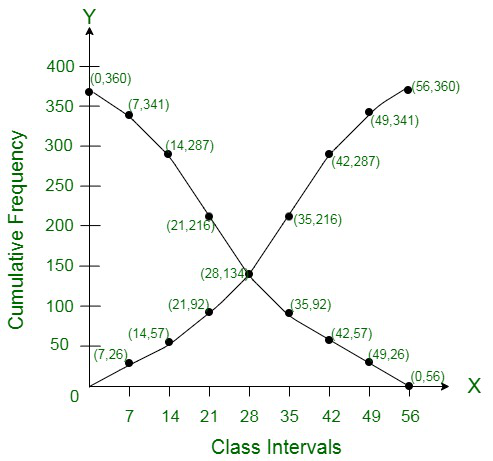Now we plot the points (7, 26), (14, 57), (21, 92), (28, 134), (35, 216), (42, 287), (49, 341), (56, 360) on the graph and join then in a frequency curve which is ‘less than ogive’

(ii) More than ogive:

First we prepare ‘more than’ frequency table as shown given below:

Now we plot the points (0, 360), (7, 341), (14, 287), (21, 216), (28, 134), (35, 92), (42, 57), (49, 26), (56, 0) on the graph and join them in free hand curve to get more than ogive.

### Question 10. The annual profits earned by 30 shops of a shopping complex in a locality give rise to the following distribution:

Draw both ogives for the above data and hence obtain the median.

Solution:

Now plot the points (5, 30), (10, 28), (15, 16), (20, 14), (25, 10), (30, 7) and (35, 3) on the graph and join them to get a more than curve.

Less than curve: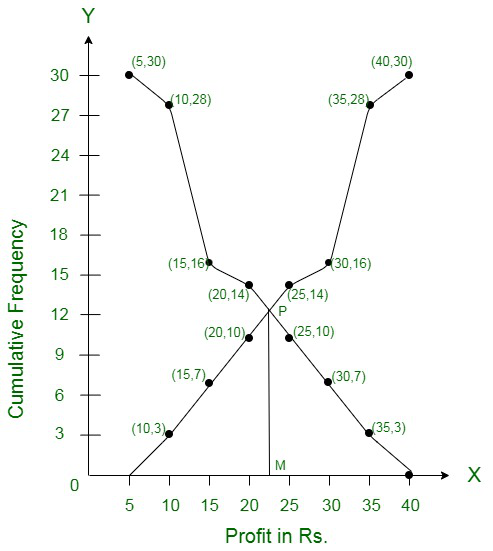Now plot the points (10, 3), (15, 7), (20, 10), (25, 14), (30, 16), (35, 28) and (40, 30) on the graph and join them to get a less them ogive. The two curved intersect at P. From P, draw PM 1 x-axis, M is the

median which is 22.5

∴ Median = Rs. 22.5 lakh

My Personal Notes arrow_drop_up
Related Tutorials